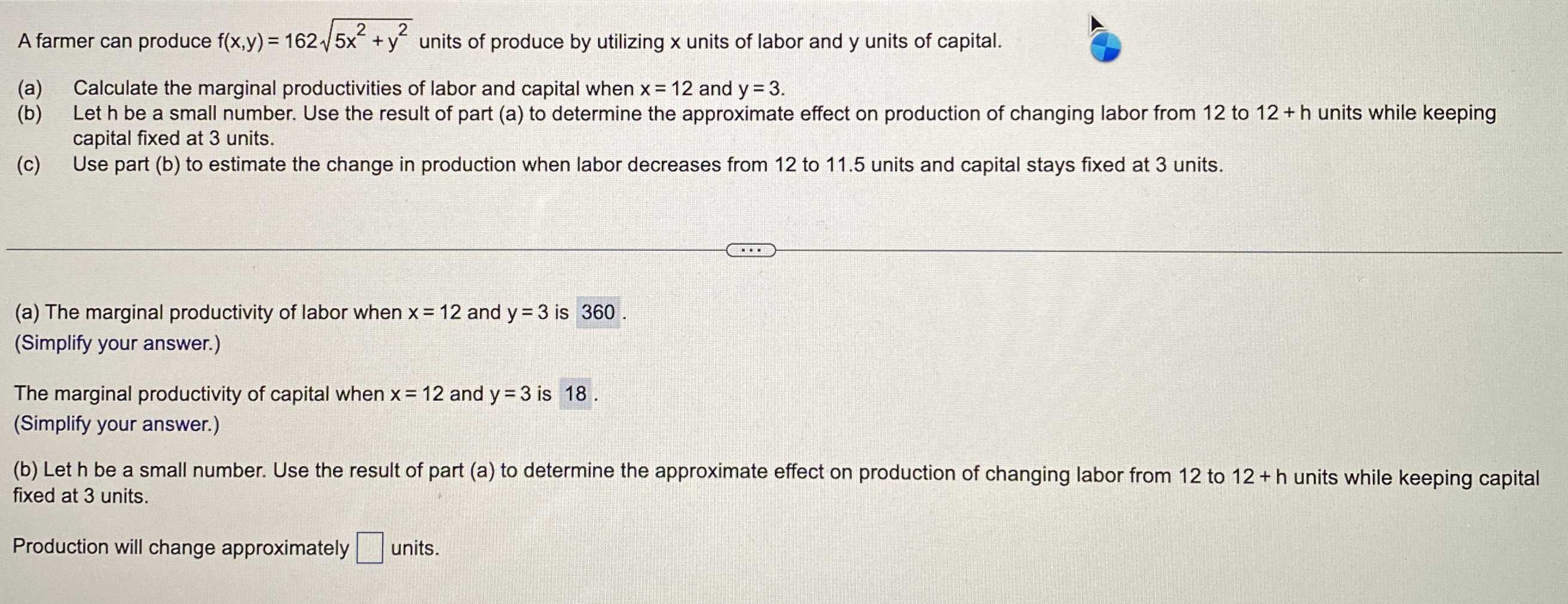### ¿Todavía tienes preguntas de matemáticas?

Pregunte a nuestros tutores expertos
Algebra
PreguntaA farmer can produce $$f ( x , y ) = 162 \sqrt { 5 x ^ { 2 } + y ^ { 2 } }$$ units of produce by utilizing $$x$$ units of labor and $$y$$ units of capital. (a) Calculate the marginal productivities of labor and capital when $$x = 12$$ and $$y = 3$$ . (b) Let $$h$$ be a small number. Use the result of part (a) to determine the approximate effect on production of changing labor from $$12$$ to $$12 + h$$ units while keeping capital fixed at $$3$$ units. (c) Use part (b) to estimate the change in production when labor decreases from $$12$$ to $$11.5$$ units and capital stays fixed at $$3$$ units. (a) The marginal productivity of labor when $$x = 12$$ and $$y = 3$$ is $$360$$ . (Simplify your answer.) The marginal productivity of capital when $$x = 12$$ and $$y = 3$$ is $$18$$ . (Simplify your answer.) (b) Let $$h$$ be a small number. Use the result of part (a) to determine the approximate effect on production of changing labor from $$12$$ to $$12 + h$$ units while keeping capital fixed at $$3$$ units. Production will change approximately $$\square$$ units.